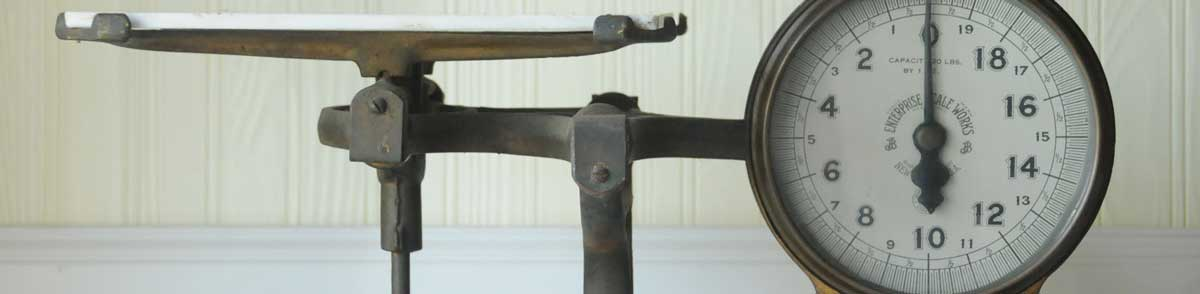# Milliliters to Kilograms Conversion Calculator

Enter the volume in milliliters below to calculate the weight in kilograms.

Results in Kilograms:1 ml = 0.001 kg

## How to Convert Milliliters to KilogramsTo convert a milliliter measurement to a kilogram measurement, divide the volume multiplied by the density of the ingredient or material by 1,000.

You can use this simple formula to convert:

kilograms = milliliters × ingredient density1,000

Thus, the volume in kilograms is equal to the milliliters times the density of the ingredient or material divided by 1,000.

For example, here's how to convert 500 milliliters to kilograms for an ingredient with a density of 0.7 g/mL.
500 ml = 500 × 0.71,000 = 0.35 kg

Milliliters and kilograms are both commonly used to measure cooking ingredients.

For cooking applications, most chefs suggest measuring dry ingredients by weight rather than volume to improve accuracy in the measurements. The density of dry ingredients can vary for a variety of reasons, such as compaction.

The best way to ensure an accurate conversion is to use a scale. When a scale is not available, a calculator like the one above is a good way to estimate the volume to weight conversion.

## Milliliters

The milliliter is a unit of volume equal to 1 cubic centimeter, 1/1,000 of a liter, or about 0.061 cubic inches.

The milliliter is an SI unit of volume in the metric system. In the metric system, "milli" is the prefix for 10-3. A milliliter is sometimes also referred to as a millilitre. Milliliters can be abbreviated as ml, and are also sometimes abbreviated as mL or mℓ. For example, 1 milliliter can be written as 1 ml, 1 mL, or 1 mℓ.

Milliliters are sometimes expressed using the abbreviation cc for medical dosage, which is the abbreviation for the cubic centimeter.

## Kilograms

The definition of the kilogram changed in 2019. One kilogram was previously equal to the mass of the platinum-iridium bar, known as the International Prototype of the Kilogram, which was stored in Sèvres, France.

The 2019 SI brochure now defines the kilogram using the Planck constant, and it is defined using the meter and second. It is roughly equal to the mass of 1,000 cubic centimeters of water.

The kilogram, or kilogramme, is the SI base unit for weight and is also a multiple of the gram. In the metric system, "kilo" is the prefix for 103. Kilograms can be abbreviated as kg; for example, 1 kilogram can be written as 1 kg.

## Milliliter to Kilogram Conversion Table

Milliliter measurements converted to kilograms for commonly used ingredients.
Volume in Milliliters: Weight in Kilograms of:
Water Milk Cooking Oil All Purpose Flour Granulated Sugar
10 ml 0.01 kg 0.0104 kg 0.0088 kg 0.00529 kg 0.007 kg
20 ml 0.02 kg 0.0208 kg 0.0176 kg 0.01058 kg 0.014 kg
30 ml 0.03 kg 0.0312 kg 0.0264 kg 0.01587 kg 0.021 kg
40 ml 0.04 kg 0.0416 kg 0.0352 kg 0.02116 kg 0.028 kg
50 ml 0.05 kg 0.052 kg 0.044 kg 0.02645 kg 0.035 kg
60 ml 0.06 kg 0.0624 kg 0.0528 kg 0.03174 kg 0.042 kg
70 ml 0.07 kg 0.0728 kg 0.0616 kg 0.03703 kg 0.049 kg
80 ml 0.08 kg 0.0832 kg 0.0704 kg 0.04232 kg 0.056 kg
90 ml 0.09 kg 0.0936 kg 0.0792 kg 0.04761 kg 0.063 kg
100 ml 0.1 kg 0.104 kg 0.088 kg 0.0529 kg 0.07 kg
110 ml 0.11 kg 0.1144 kg 0.0968 kg 0.05819 kg 0.077 kg
120 ml 0.12 kg 0.1248 kg 0.1056 kg 0.06348 kg 0.084 kg
130 ml 0.13 kg 0.1352 kg 0.1144 kg 0.06877 kg 0.091 kg
140 ml 0.14 kg 0.1456 kg 0.1232 kg 0.07406 kg 0.098 kg
150 ml 0.15 kg 0.156 kg 0.132 kg 0.07935 kg 0.105 kg
160 ml 0.16 kg 0.1664 kg 0.1408 kg 0.08464 kg 0.112 kg
170 ml 0.17 kg 0.1768 kg 0.1496 kg 0.08993 kg 0.119 kg
180 ml 0.18 kg 0.1872 kg 0.1584 kg 0.09522 kg 0.126 kg
190 ml 0.19 kg 0.1976 kg 0.1672 kg 0.10051 kg 0.133 kg
200 ml 0.2 kg 0.208 kg 0.176 kg 0.1058 kg 0.14 kg
210 ml 0.21 kg 0.2184 kg 0.1848 kg 0.11109 kg 0.147 kg
220 ml 0.22 kg 0.2288 kg 0.1936 kg 0.11638 kg 0.154 kg
230 ml 0.23 kg 0.2392 kg 0.2024 kg 0.12167 kg 0.161 kg
240 ml 0.24 kg 0.2496 kg 0.2112 kg 0.12696 kg 0.168 kg
250 ml 0.25 kg 0.26 kg 0.22 kg 0.13225 kg 0.175 kg
260 ml 0.26 kg 0.2704 kg 0.2288 kg 0.13754 kg 0.182 kg
270 ml 0.27 kg 0.2808 kg 0.2376 kg 0.14283 kg 0.189 kg
280 ml 0.28 kg 0.2912 kg 0.2464 kg 0.14812 kg 0.196 kg
290 ml 0.29 kg 0.3016 kg 0.2552 kg 0.15341 kg 0.203 kg
300 ml 0.3 kg 0.312 kg 0.264 kg 0.1587 kg 0.21 kg
310 ml 0.31 kg 0.3224 kg 0.2728 kg 0.16399 kg 0.217 kg
320 ml 0.32 kg 0.3328 kg 0.2816 kg 0.16928 kg 0.224 kg
330 ml 0.33 kg 0.3432 kg 0.2904 kg 0.17457 kg 0.231 kg
340 ml 0.34 kg 0.3536 kg 0.2992 kg 0.17986 kg 0.238 kg
350 ml 0.35 kg 0.364 kg 0.308 kg 0.18515 kg 0.245 kg
360 ml 0.36 kg 0.3744 kg 0.3168 kg 0.19044 kg 0.252 kg
370 ml 0.37 kg 0.3848 kg 0.3256 kg 0.19573 kg 0.259 kg
380 ml 0.38 kg 0.3952 kg 0.3344 kg 0.20102 kg 0.266 kg
390 ml 0.39 kg 0.4056 kg 0.3432 kg 0.20631 kg 0.273 kg
400 ml 0.4 kg 0.416 kg 0.352 kg 0.2116 kg 0.28 kg

## References

1. National Institute of Standards & Technology, Metric Cooking Resources, https://www.nist.gov/pml/weights-and-measures/metric-cooking-resources
2. National Institute of Standards and Technology, Specifications, Tolerances, and Other Technical Requirements for Weighing and Measuring Devices, Handbook 44 - 2019 Edition, https://nvlpubs.nist.gov/nistpubs/hb/2019/NIST.HB.44-2019.pdf
3. International Bureau of Weights and Measures, The International System of Units, 9th Edition, 2019, https://www.bipm.org/documents/20126/41483022/SI-Brochure-9-EN.pdf